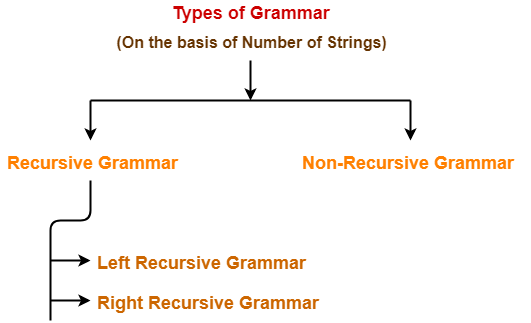## Grammar in Automata-

Before you go through this article, make sure that you have gone through the previous article on Types of Grammar in Automata.

On the basis of number of strings, grammars are classified as-1. Recursive Grammar
2. Non-Recursive Grammar

## 1. Recursive Grammar-

• A grammar is said to be recursive if it contains at least one production that has the same variable at both its LHS and RHS.

OR

• A grammar is said to be recursive if and only if it generates infinite number of strings.

A recursive grammar may be either-

1. Left recursive grammar
2. Right recursive grammar

### A) Left Recursive Grammar-

• A recursive grammar is said to be left recursive if the leftmost variable of RHS is same as variable of LHS.

OR

• A recursive grammar is said to be left recursive if it has Left Recursion.

### Example-

S → Sa / b

(Left Recursive Grammar)

### B) Right Recursive Grammar-

• A recursive grammar is said to be right recursive if the rightmost variable of RHS is same as variable of LHS.

OR

• A recursive grammar is said to be right recursive if it has right recursion.

### Example-

S → aS / b

(Right Recursive Grammar)

## 2. Non-Recursive Grammar-

• A grammar is said to be non-recursive if it contains no production that has the same variable at both its LHS and RHS.

OR

• A grammar is said to be non-recursive if and only if it generates finite number of strings.

### NOTE

A non-recursive grammar has neither left recursion nor right recursion.

### Example-

S → aA / bB

A → a / b

B → c / d

(Non-Recursive Grammar)

The language generated from this grammar is L = { aa , ab , bc , bd }

Since the grammar generates finite number of strings, therefore it is a non-recursive grammar.

## Important Notes-

### Note-01:

The grammar which is either left recursive or right recursive is always unambiguous.

Examples-

• S → aS / b   (Unambiguous Grammar)
• S → Sa / b   (Unambiguous Grammar)

### Note-02:

The grammar which is both left recursive and right recursive is always ambiguous.

Example-

E → E + E / E – E / E x E / id

(Ambiguous Grammar)

### Note-03:

• Left recursive grammar is not suitable for Top down parsers.
• This is because it makes the parser enter into an infinite loop.
• To avoid this situation, it is converted into its equivalent right recursive grammar.
• This is done by eliminating left recursion from the left recursive grammar.

### Note-04:

• The conversion of left recursive grammar into right recursive grammar and vice-versa is decidable.

To gain better understanding about Recursive Grammar,

Watch this Video Lecture

Next Article- Ambiguous Vs Unambiguous Grammar

Get more notes and other study material of Theory of Automata and Computation.

Watch video lectures by visiting our YouTube channel LearnVidFun.